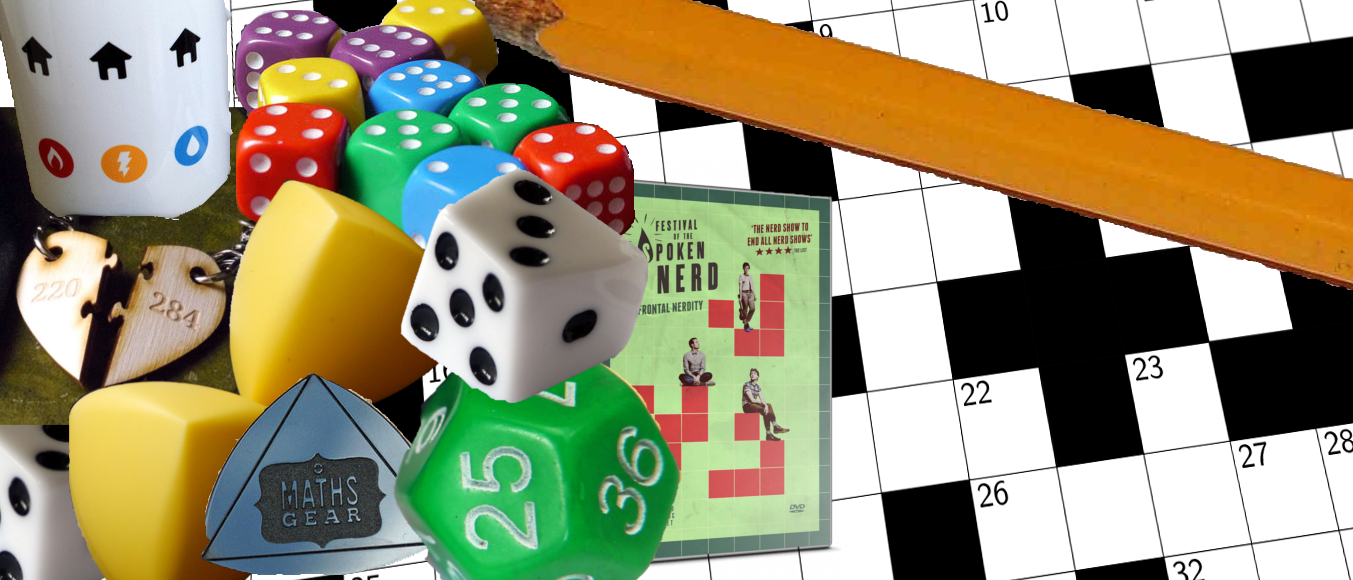# Prize crossnumber, Issue 06

Win £100 of Maths Gear goodies by solving our famously fiendish crossnumberOur original prize crossnumber is featured on pages 52 and 53 of Issue 06.

Correction: The pdf was incorrect and 5D did not match the clues below. This has now been fixed.
Clarification: Added brackets to 29A and 34D to reduce ambiguity.

### Rules

• Although many of the clues have multiple answers, there is only one solution to the completed crossnumber. As usual, no numbers begin with 0. Use of Python, OEIS, Wikipedia, etc. is advised for some of the clues.
• One randomly selected correct answer will win a £100 Maths Gear goody bag. Three randomly selected runners up will win a Chalkdust t-shirt. The prizes have been provided by Maths Gear, a website that sells nerdy things worldwide, with free UK shipping. Find out more at mathsgear.co.uk
• To enter, submit the sum of the across clues via this form by 8 January 2018. Only one entry per person will be accepted. Winners will be notified by email and announced on our blog by 22 January 2018.

### Crossnumber

Crossnumber #6, set by Humbug:

### Clues

#### Across

• 1. This number is divisible by both the sum and the product of its digits. (2)
• 3. All the digits of this number are different. (4)
• 5. The product of 1A and 5D. (3)
• 6. The difference between 22D and 23D. (5)
• 11. A prime number. (2)
• 12. A square number. (2)
• 13. The maximum number of pieces a cube can be cut into with 36 planar cuts. (4)
• 15. A factor of 7D. (4)
• 17. When written in hexadecimal, this number spells a common English word. (4)
• 18. This number and 19D are a pair of triangle numbers whose sum and difference are also triangle numbers. (2)
• 20. A palindrome. (5)
• 23. A multiple of 7 whose digits add to 7. (4)
• 24. A factor of 33D. (2)
• 25. The largest number whose square has 12 digits. (6)
• 29. A factor of (12 more than 22D). (2)
• 30. A multiple of 26D. (3)
• 31. The sum of the digits of 29A. (2)
• 32. The product of 36D and 12A. (3)
• 33. A Fibonacci number. (2)
• 37. A palindrome that is a multiple of 1111. (7)
• 41. An odd number. (2)
• 42. Each digit of this number is one more than the previous digit. (4)
• 43. This number and 29D are a pair of triangle numbers whose sum and difference are also triangle numbers. (3)
• 44. The mean of 45D and 41A. (2)
• 46. This number and 47A are a pair of triangle numbers whose sum and difference are also triangle numbers. (7)
• 47. see 46A. (7)

#### Down

• 1. Take this number’s digits as the first two numbers in a sequence. Form a Fibonacci-like sequence. This number is in this sequence. (2)
• 2. This number is divisible by both the sum and the product of its digits. (2)
• 4. The square root of 3A. (2)
• 5. The product of the first two digits of 35D. (2)
• 7. Not a prime number. (5)
• 8. Ten times the sum of the across clues in this crossnumber. (8)
• 9. A factor of 45D. (2)
• 10. The product of 11A and 12A. (3)
• 11. Three more than a multiple of four. (3)
• 14. A multiple of 45D. (7)
• 16. The product of 11A and 36D. (3)
• 19. see 18A. (2)
• 21. A factor of 20A. (2)
• 22. A multiple of 36D. (6)
• 23. A multiple of 44A and 28D. (6)
• 24. An integer $x$ that satisfies $x^2+1=2y^4$, for some integer $y$. (3)
• 26. Not equal to 44A. (2)
• 27. The price of a £100 coat after discounting 10% then adding 10% tax. (2)
• 28. The price of a £100 coat after adding 10% tax then discounting 10%. (2)
• 29. see 43A. (3)
• 33. A multiple of 17A. (5)
• 34. A multiple of (one more than 15A). (5)
• 35. An even number. (3)
• 36. The mean of 11A, 12A and 36D. (2)
• 37. The sum of the digits of this number’s cube, sixth power and seventh power are all equal. (2)
• 38. In a base other than 10, this number can be written as 110001. (4)
• 39. The product of the digits of 34D. (2)
• 40. This number is equal to the product of its digits plus the sum of its digits. (2)
• 45. A multiple of 5. (3)